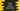# Java 8 example to convert a string to integer stream (IntStream)### Java 8 example to convert a string to integer stream :

In this tutorial, we will learn how to convert a string to IntStream. We will use chars() method to convert a string to IntStream. To print out the result of the IntStream, we will use Stream.forEach method. Then we will pass one lambda expression to print out the characters.

Let’s take a look at the program first :

### Java program :

``````import java.util.stream.IntStream;

public class Main {

public static void main(String[] args) {
//1
String sampleString = "This is a sample String";

//2
IntStream stream = sampleString.chars();

//3
stream.forEach(element -> System.out.println(((char)element)));
}

}``````

### Explanation :

The commented numbers in the above program denote the step number below :

1. The string sampleString is the string we are going to convert.
2. Using chars(), we have converted it to IntStream.
3. Using forEach, we have printed out the char value of each value of the IntStream.

### Output :

``````T
h
i
s

i
s

a

s
a
m
p
l
e

S
t
r
i
n
g``````

We have used println to print each character on a different line. We can also use print to print the characters in one line.

``````import java.util.stream.IntStream;

public class Main {

public static void main(String[] args) {
String sampleString = "This is a sample String";

IntStream stream = sampleString.chars();

stream.forEach(element -> System.out.print(((char)element)));
}

}``````

It will print :

``This is a sample String``